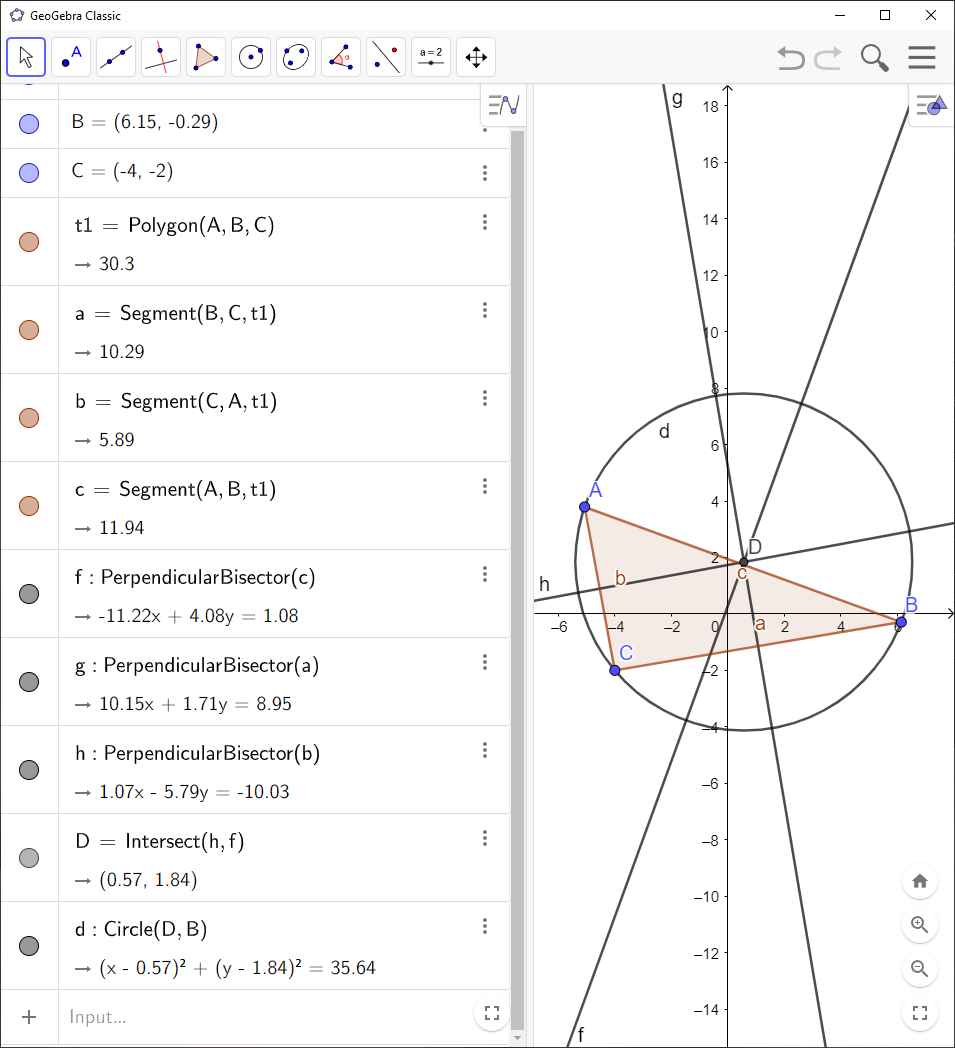# How to Find Circumcircle and Circumcenter with GeoGebra

You can use `GeoGebra` to find circumcircle and circumcenter of a triangle:

`GeoGebra` Instruction 1

1.
Open `Algebra View` and `Graphics View` under`View` in`Menu`.
2.
Make a triangle with the vertices $A$, $B$ and $C$ by clicking `Polygon`. Then you need to click on three positions in `Graphics View` where you want the vertices of the triangle to be. Finally, complete the triangle by clicking on the point that you started with.
3.
Click on `Perpendicular Bisector`by first clicking tool number 4, then tool number 3 in the drop-down list.
4.
Construct the perpendicular bisector of $AB$, the perpendicular bisector of $BC$ and the perpendicular bisector of $AC$ by clicking on the sides of $△ABC$.
5.
Click `Intersect`by first clicking tool number 4, then tool number 4 in the drop-down list, and use it to find the point of intersection $D$ of two perpendicular bisectors. The point $D$ is the circumcenter.
6.
Click `Circle with Center through Point`to construct a circle with the circumcenter as the center, and one of the vertices of the triangle as the point. You will see that the circle actually touches all vertices even though you just clicked one corner. You’ve drawn the circumcircle!